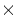Next: Reserved Keywords for ASCII Up: ASCII Table Extension Previous: ASCII Table Extension

3.4.1 Required Keywords for ASCII Table Extension

The following keywords are required for ASCII table extensions and must appear in the first eight card images in the following order:

1.
XTENSION (character) has the value 'TABLE ' for ASCII table extensions.
2.
BITPIX (integer) must have the value of 8, indicating printable ASCII characters.

3.
NAXIS (integer) must have the value of 2 for ASCII table extension headers. The axes are the rows and columns of the table.

4.
NAXIS1 (integer) gives the number of characters in a table row.

5.
NAXIS2 (integer) gives the number of rows in the table. The value can be 1. While a value of zero is permitted, that value would indicate that no table is present.

6.
PCOUNT (integer) is required with the value of 0 for the ASCII table extension.

7.
GCOUNT (integer) is required with the value of 1 for the ASCII table extension. Each extension contains one table; the total number of bytes is NAXIS1NAXIS2.

8.
TFIELDS (integer) has a value that gives the number of fields in each table row. Values from 0 to 999 are allowed.

Note that while the rules for generalized extensions do not specify where the PCOUNT and GCOUNT keywords appear between the last NAXISn keyword and the END keyword, the ASCII table extension requires that they appear immediately after the NAXIS2 keyword.

The following required keywords may appear anywhere between the TFIELDS and the END keywords.

• TBCOLn (integer) is the column number of the first character in field n. The first column of a row is numbered 1.
• TFORMn (character) is the FORTRAN format of field n (I, A, E, D, F). To repeat a field, the format must be repeated using separate TFORMn and TBCOLn card images; the repeat count (e.g., 2Fx.x) of FORTRAN cannot be used. The TFORMn values specify the width of the field and are of the form Iw, Aw, Fw.d, Ew.dEe, or Dw.dEe (integers, characters, single precision floating point, single precision exponential, and double precision exponential, respectively). The w is the width of the field, d the number of digits after the decimal point, and e is the number of digits in the exponent, as in FORTRAN. For integer fields, if -0 needs to be distinguished from +0 (e.g., degrees of declination), the sign will have to be a separate character field. It is better to use floating point. TBCOLn and TFORMn keywords must be present for values of n from 1 to the value of TFIELDS.

END is always the last keyword; the remainder of the header record is filled with ASCII blanks.Next: Reserved Keywords for ASCII Up: ASCII Table Extension Previous: ASCII Table Extension